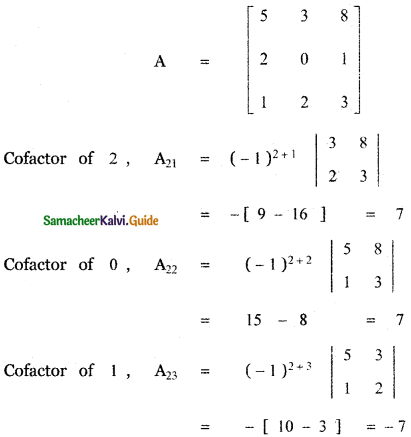Tamilnadu State Board New Syllabus Samacheer Kalvi 11th Maths Guide Pdf Chapter 7 Matrices and Determinants Ex 7.2 Text Book Back Questions and Answers, Notes.

## Tamilnadu Samacheer Kalvi 11th Maths Solutions Chapter 7 Matrices and Determinants Ex 7.2

Question 1.
without expanding the determinant,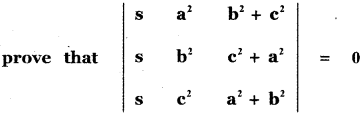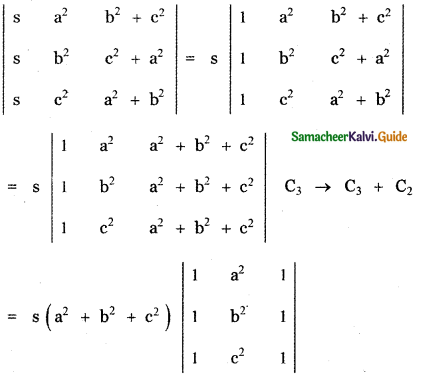= s (a2 + b2 + c2) × 0
since two columns are equal.
= 0Question 2.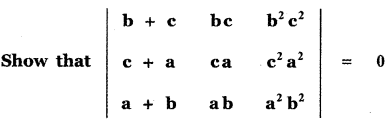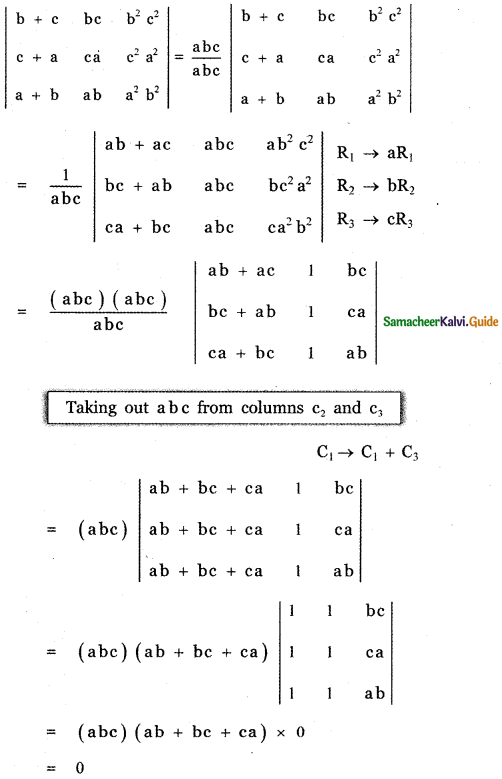Question 3.
Prove that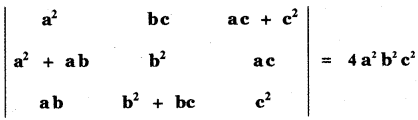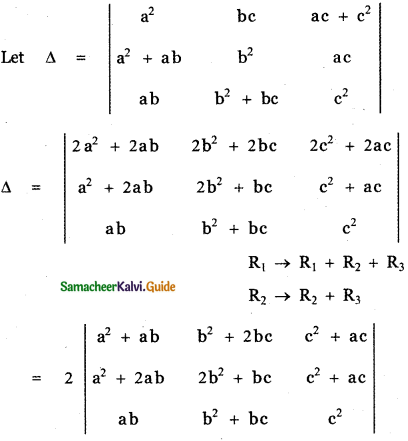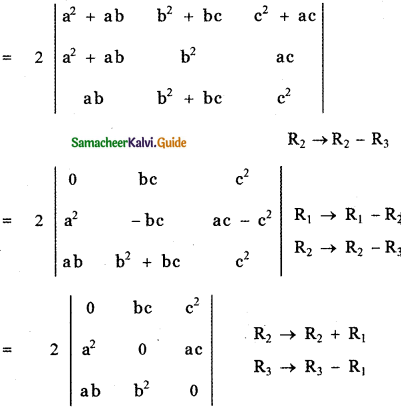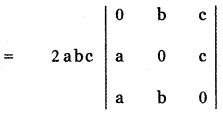= 2abc [0 – b(0 – ac) + c(ab – 0)]
= 2 abc [ abc + abc ]
= 2 abc × 2abc
Δ = 4 a2b2c2Question 4.
Prove that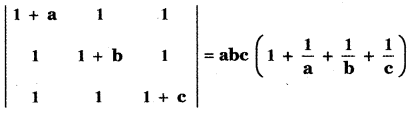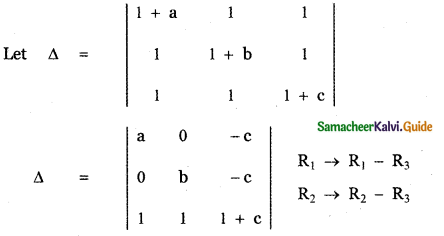= a [b(1 + c) + c (1)] – 0 – c [0 – b]
= a[b + bc + c] + bc
= ab + abc + ac + bc
= abc + ab + bc + ac
= abc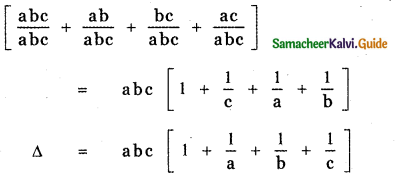Question 5.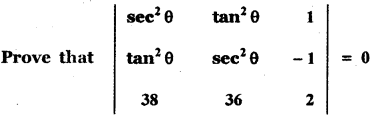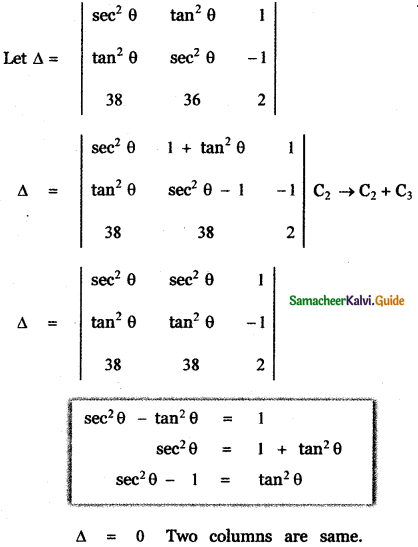Question 6.
show that $$\left| \begin{matrix} x\quad +\quad 2a & y\quad +\quad 2b & z\quad +\quad 2c \\ x & y & z \\ a & b & c \end{matrix} \right|$$ = 0
Let Δ = $$\left| \begin{matrix} x\quad +\quad 2a & y\quad +\quad 2b & z\quad +\quad 2c \\ x & y & z \\ a & b & c \end{matrix} \right|$$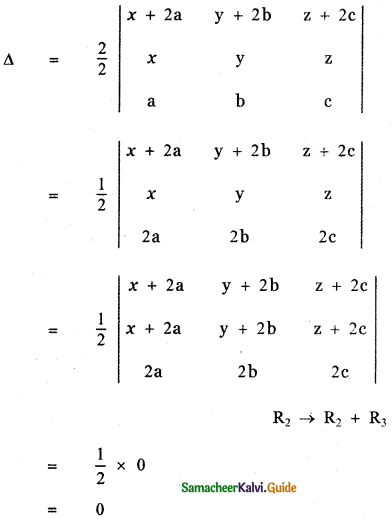Question 7.
Write the general form of a 3 × 3 skew- symmetric matrix and prove that its determinant is 0.
A square matrix A = [ aij ]3 × 3 is a skew – symmetric matrix if aij = – aij for all i,j and the elements on the main diagonal of a skew – symmetric matrix are zero.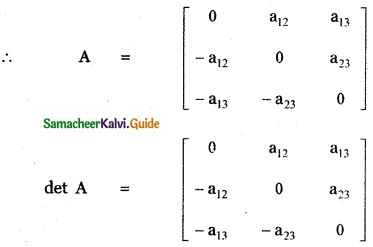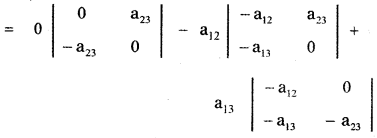= 0 – a12 (0 + a13 a23) + a13 (a12 a23 – 0)
= – a12 a13 a23 + a13 a12 a23
= 0
Hence the determinant of a skew – symmetric matrix is 0.Question 8.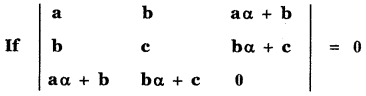Prove that a, b, c are in G. P or α is a root of ax2 + 2bx + c = 0.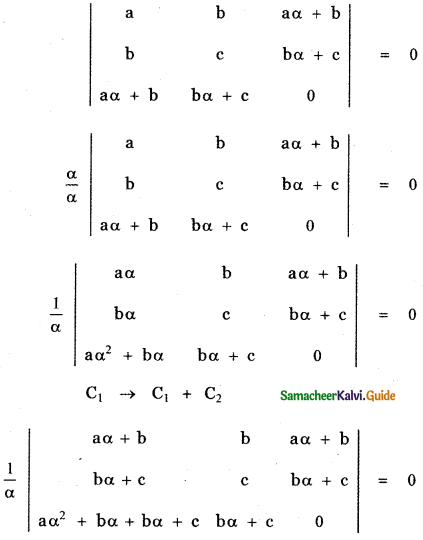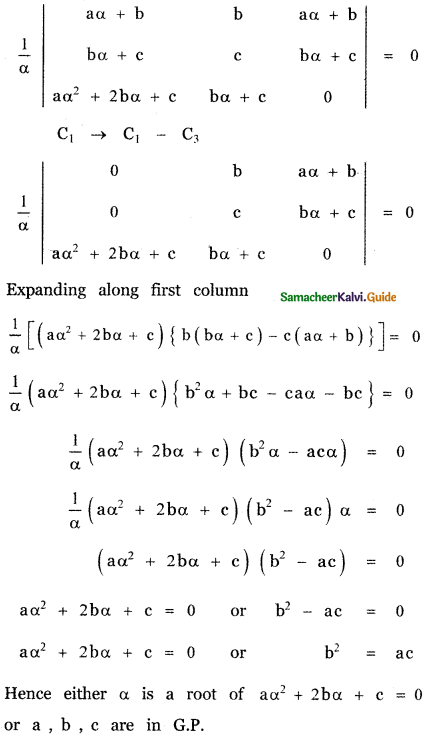Question 9.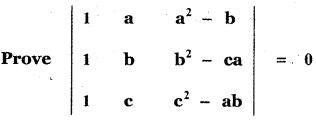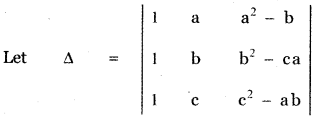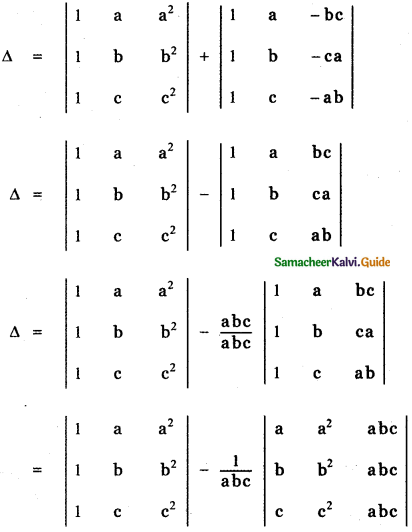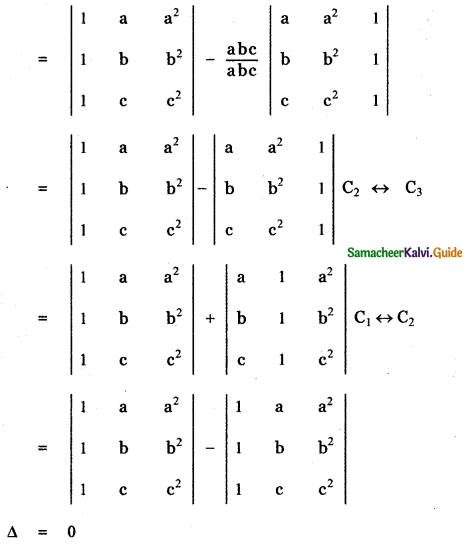Question 10.
If a, b, c are pth, qth and rth terms of an A.P, find the value of $$\left| \begin{matrix} a & b & c \\ p & q & r \\ 1 & 1 & 1 \end{matrix} \right|$$
Given a, b, c are pth, qth and rth terms of an A.P.
tp = a = A + (p – 1)D,
tq = b = A + (q – 1)D,
tr = c = A + (r – 1) D
where A – first term , D – Common difference of the AP.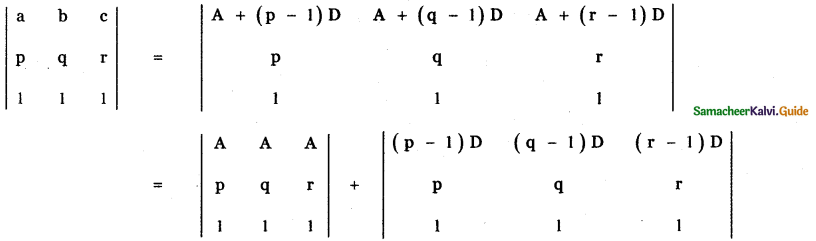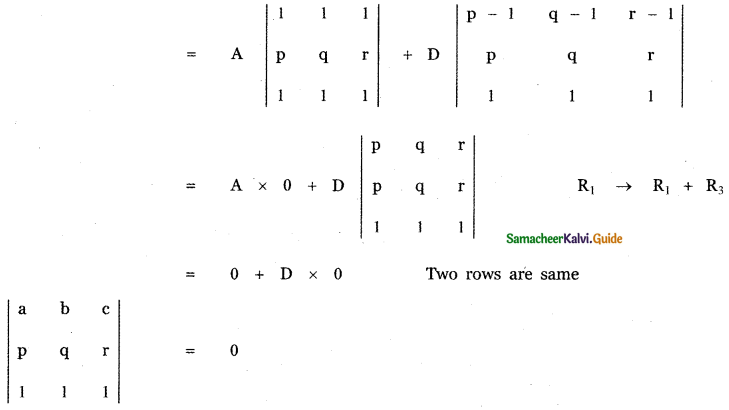Question 11.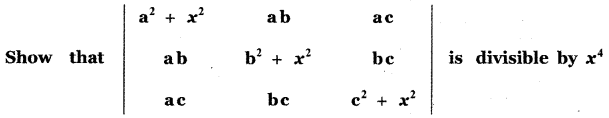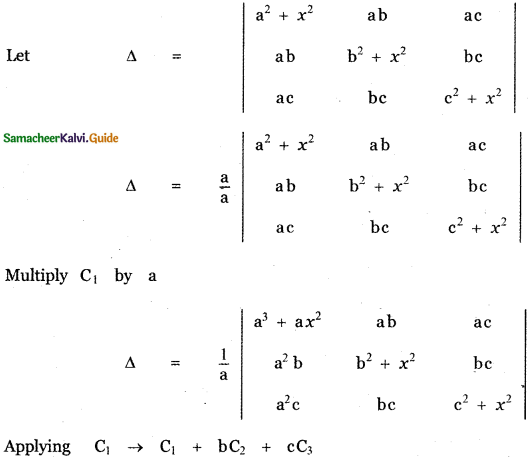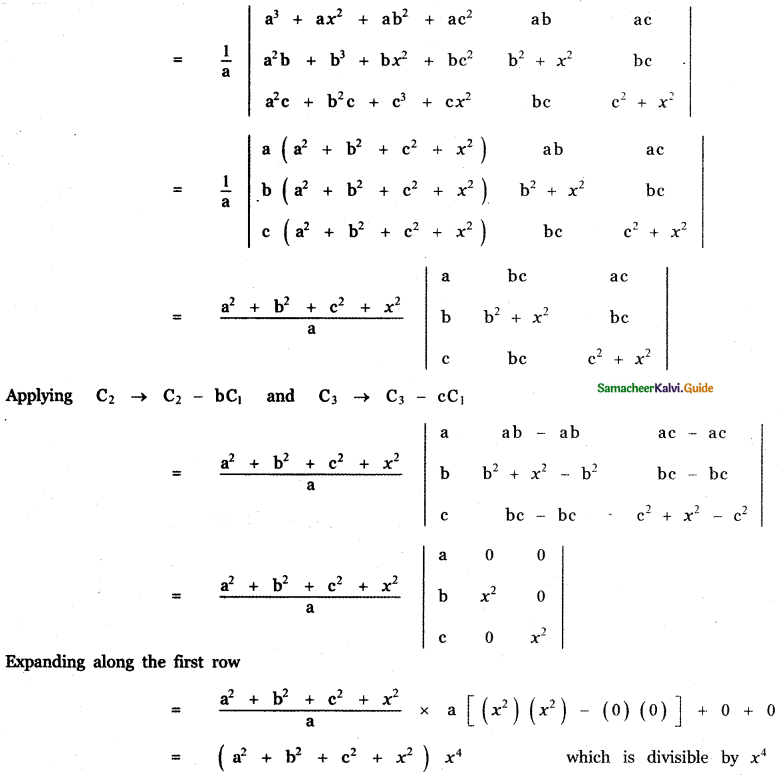Question 12.
If a , b , c , are all positive, and are pth, qth and rth terms of a G.P., show that $$\left| \begin{matrix} log\quad a & p & 1 \\ log\quad b & q & 1 \\ log\quad c & r & 1 \end{matrix} \right|$$ – 0
Given a, b, c are the pth, qth and rth terms of a G.P.
∴ a = ARp-1, b = ARq-1, c = ARr-1
where A is the first term , R – common ratio.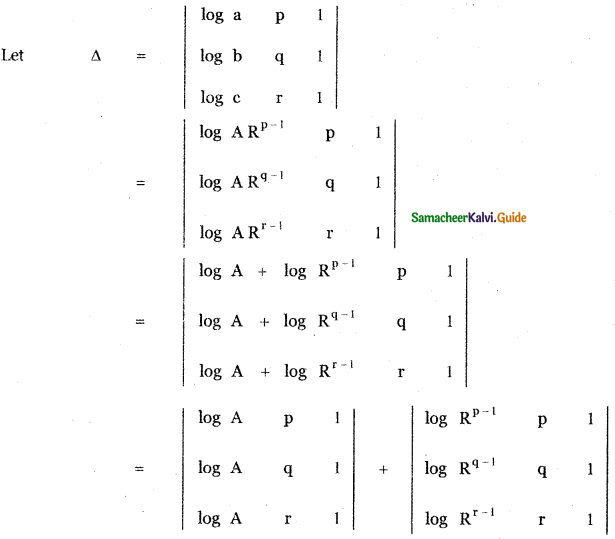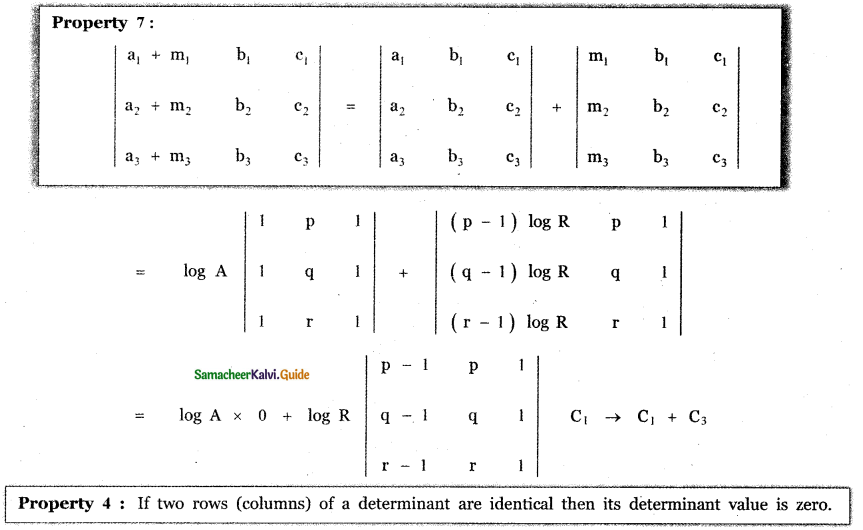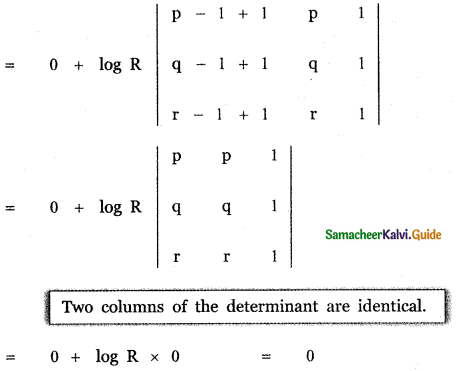Question 13.
Find the value of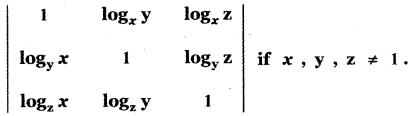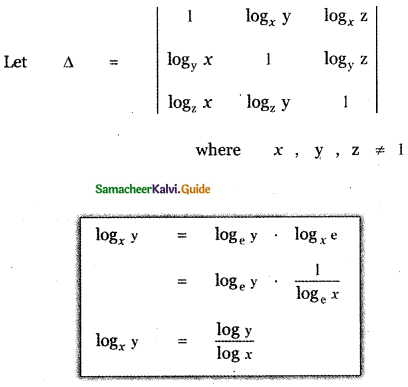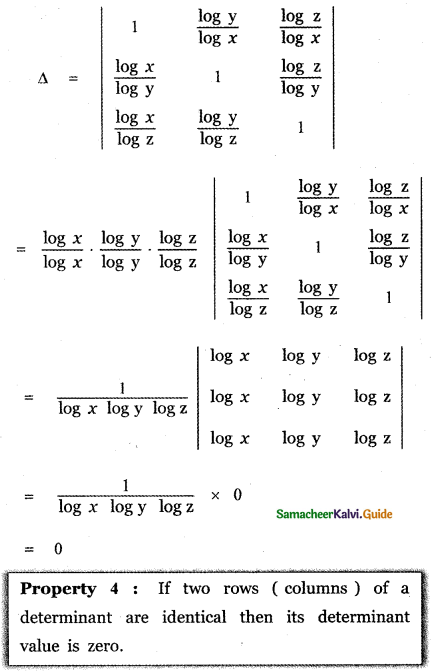Question 14.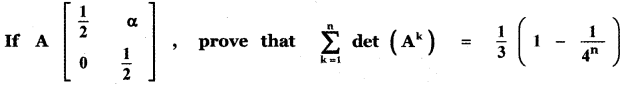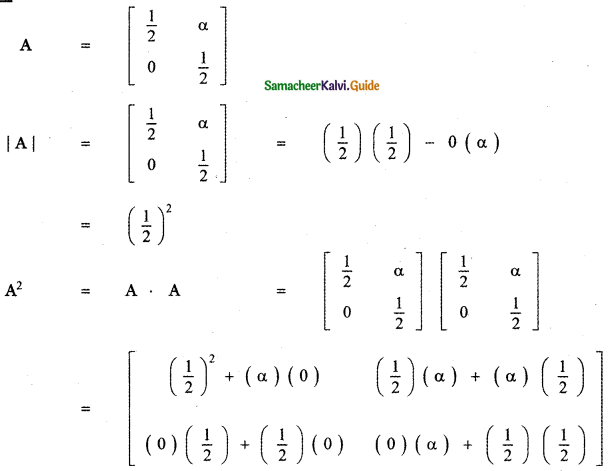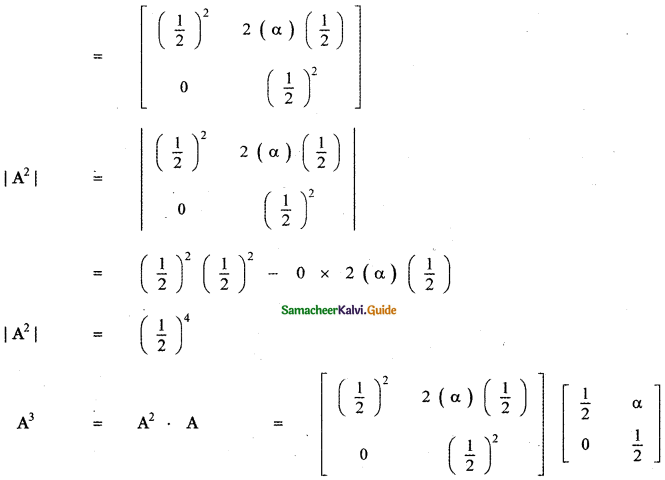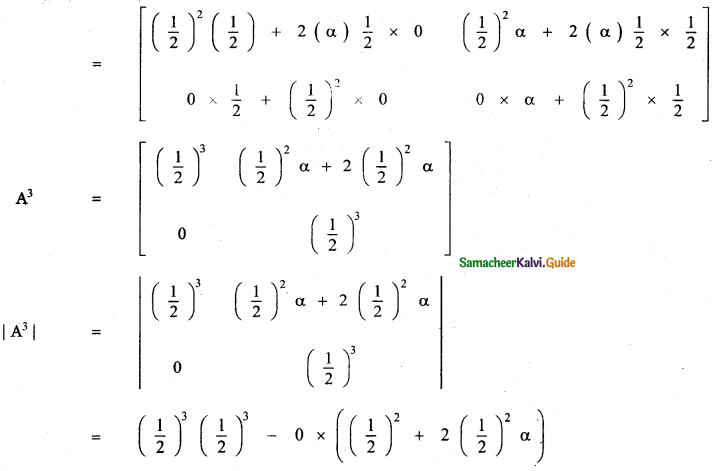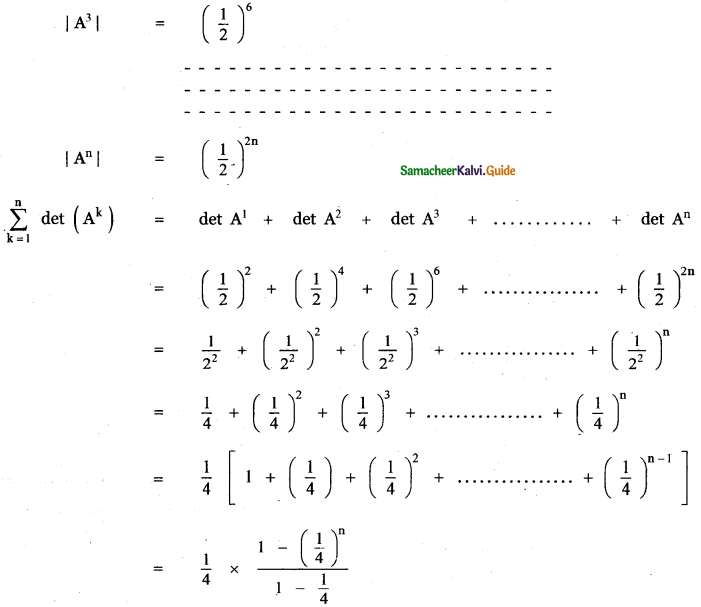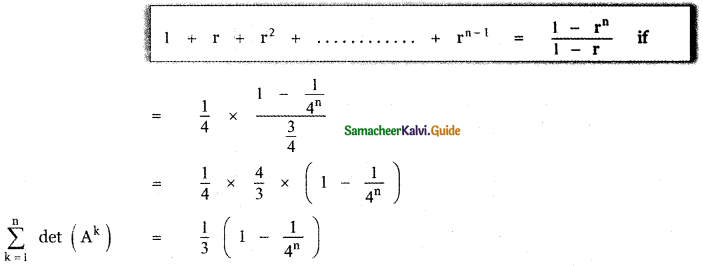Question 15.
Without expanding, evaluate the following determinants:
(i) $$\left| \begin{matrix} 2 & 3 & 4 \\ 5 & 6 & 8 \\ 6x & 9x & 12x \end{matrix} \right|$$
(ii) $$\left| \begin{matrix} x\quad +\quad y & y\quad +\quad z & z\quad +\quad x \\ z & x & y \\ 1 & 1 & 1 \end{matrix} \right|$$
(i) $$\left| \begin{matrix} 2 & 3 & 4 \\ 5 & 6 & 8 \\ 6x & 9x & 12x \end{matrix} \right|$$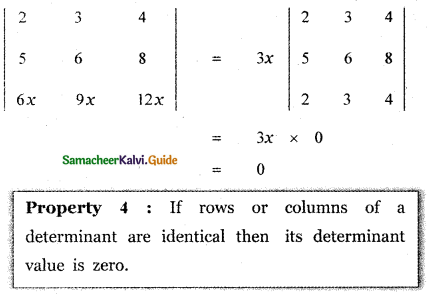(ii) $$\left| \begin{matrix} x\quad +\quad y & y\quad +\quad z & z\quad +\quad x \\ z & x & y \\ 1 & 1 & 1 \end{matrix} \right| [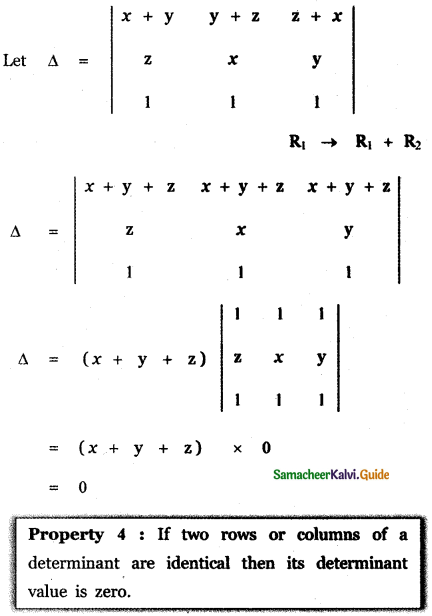Question 16. If A is a Square, matrix, and |A| = 2, find the value of |A AT|. Answer: |A| = 2 (Given) |AT| = 2 Now |AAT| = |A| |AT| = 2 × 2 = 4. Question 17. If A and B are square matrices of order 3 such that |A| = -1 and |B| = 3, find the value of |3 AB|. Answer: Given |A| = -1 : |B| = 3 Given A and B are square matrices of order 3. ∴ |kAB| = k3 |AB| Here k = 3 ∴ |3AB| = 33 |AB| = 27 |AB| = 27 (-1) (3) = -81 Question 18. If λ = – 2, determine the value of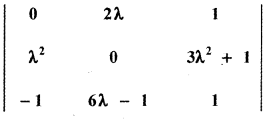Answer: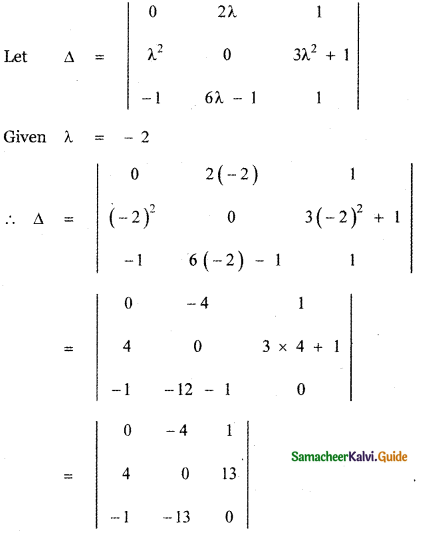Expanding along the first row Δ = 0 + 4 [4 × 0 – (- 1 ) ( 13)] + [4 × -13 – 0 × – 1] = 4 [0 + 13] + 1 [- 52 + 0] = 52 – 52 = 0Question 19. Determine the roots of the equation [latex]\left| \begin{matrix} 1 & 4 & 20 \\ 1 & -2 & 5 \\ 1 & 2x & { 5x }^{ 2 } \end{matrix} \right|$$ = 0
$$\left| \begin{matrix} 1 & 4 & 20 \\ 1 & -2 & 5 \\ 1 & 2x & { 5x }^{ 2 } \end{matrix} \right|$$ = 0 ………… (1)
Put x = -1 then (1) ⇒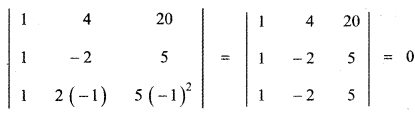∴ x = – 1 satisfies equation (1)
Hence x = – 1 is a root of equation (1)
Put x = 2 then ……….. (1)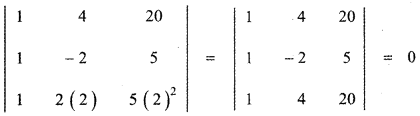[Property 4: If two rows (columns) of a determinant are identical then its determinant value is zero.]

∴ x = 2 satisfies equation (1)
Hence x = 2 is a root of equation (1)
Hence the required roots are x = -1 , 2

Question 20.
Verify that det (AB) = (det A) (det B) for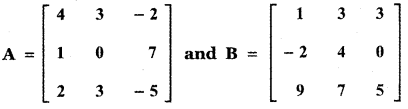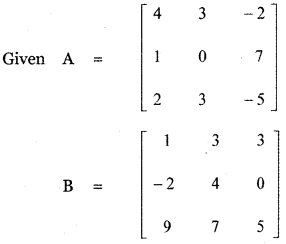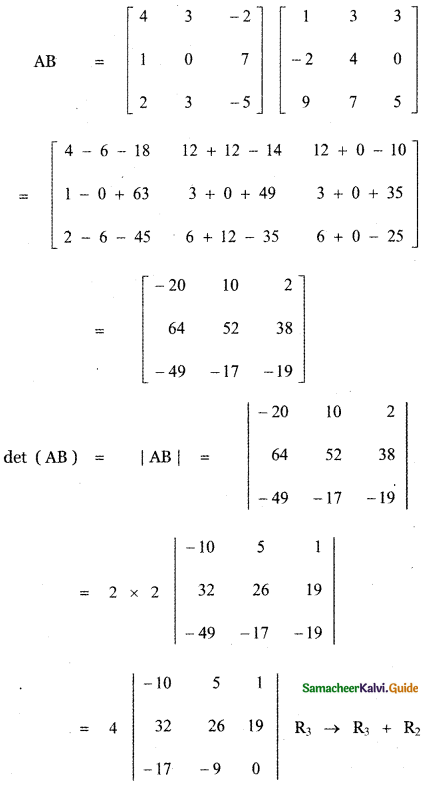= 4 [-10 (0 – 9 × 19) – 5 (0 + 17 × 19) + 1 (32 × 9 + 17 × 26)]
= 4 [1710 – 5 × 323 + 288 + 442]
= 4 [1710 – 1615 + 730]
= 4 [2440 – 1615]
= 4 × 825
det (AB) = 3300 …….. (1)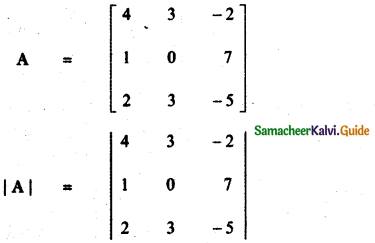= 4(0 – 21) – 3 (- 5 – 14) – 2 (3 – 0)
= -84 – 3 × – 19 – 6
= -84 + 57 – 6
= -90 + 57
det A = -33 ………… (2)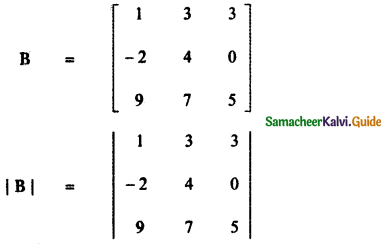= 1 (20 – 0) – 3 (- 10 – 0) + 3 (-14 – 36)
= 20 + 30 + 3 × – 50
= 50 – 150
det A = – 100 ……….. (3)

From equations (2) and (3)
(det A) (det B) = – 33 × – 100
(detA) (det B) = 3300 ………… (4)
From equations (1) and (4), we have
det (AB) = (det A) (det B)Question 21.
Using cofactors of elements of second row, evaluate |A|, where A = $$\left[ \begin{matrix} 5 & 3 & 8 \\ 2 & 0 & 1 \\ 1 & 2 & 3 \end{matrix} \right]$$